Next: Plotting functions Up: Subsidiary calculation functions Previous: stone2000Rcsp.m   Contents

## thickness.m

Syntax:

Sthick = thickness(zmax, Lambdasp, rho)


Calculates the thickness correction factor for nuclide production by spallation. The arguments are zmax, the sample thickness in cm; Lambdasp, the effective attenuation length for production by neutron spallation in atoms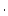g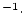yr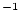; and rho, the sample density in gcm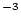. Accepts vector inputs.

Calculating the thickness correction factor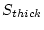begins by assuming that the production rate decreases with depth according to a single exponential function: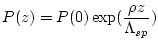(81)

where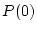is the surface production rate and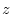is depth below the surface (cm). The thickness scaling factoris then: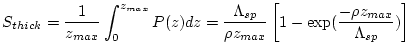(82)

where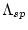is the effective attenuation length for production by spallation (input argument Lambdasp) and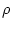is the sample density (input argument rho).

2007-11-13